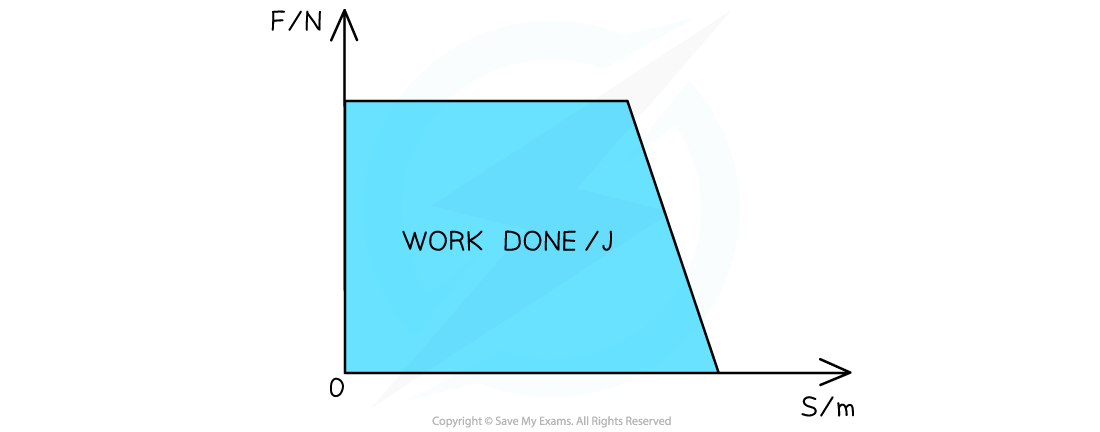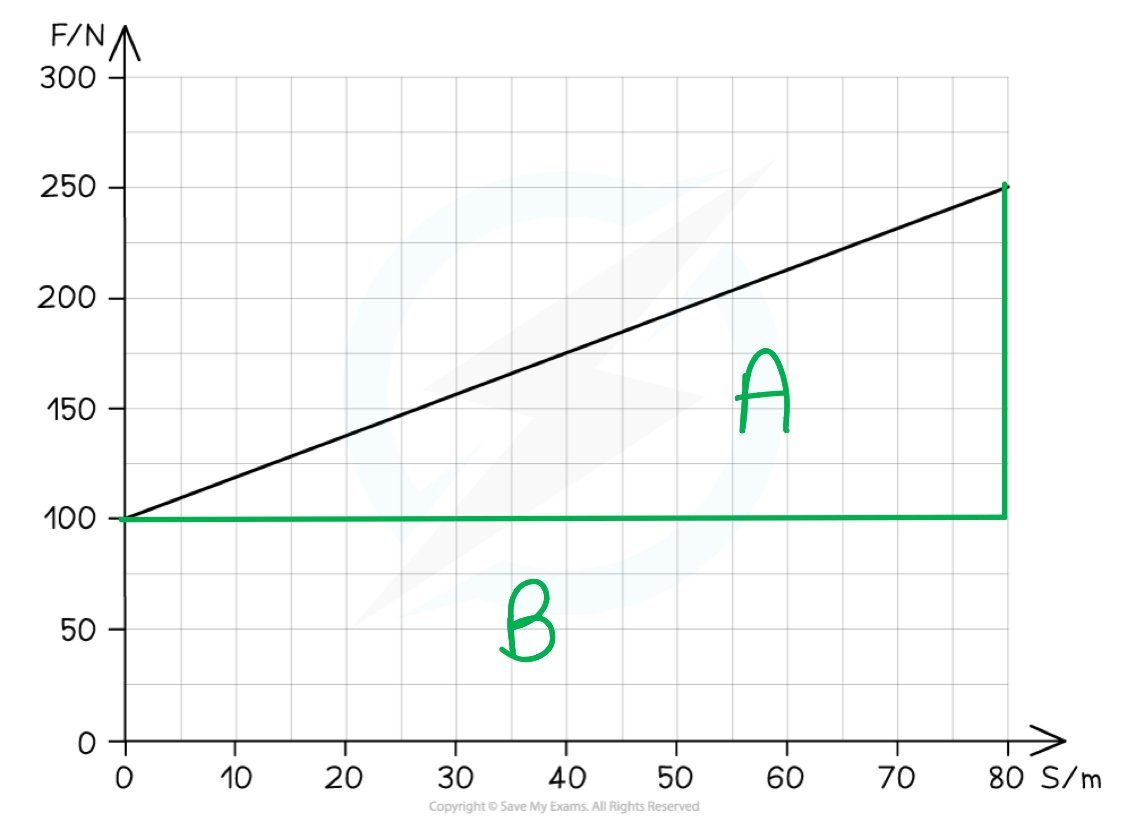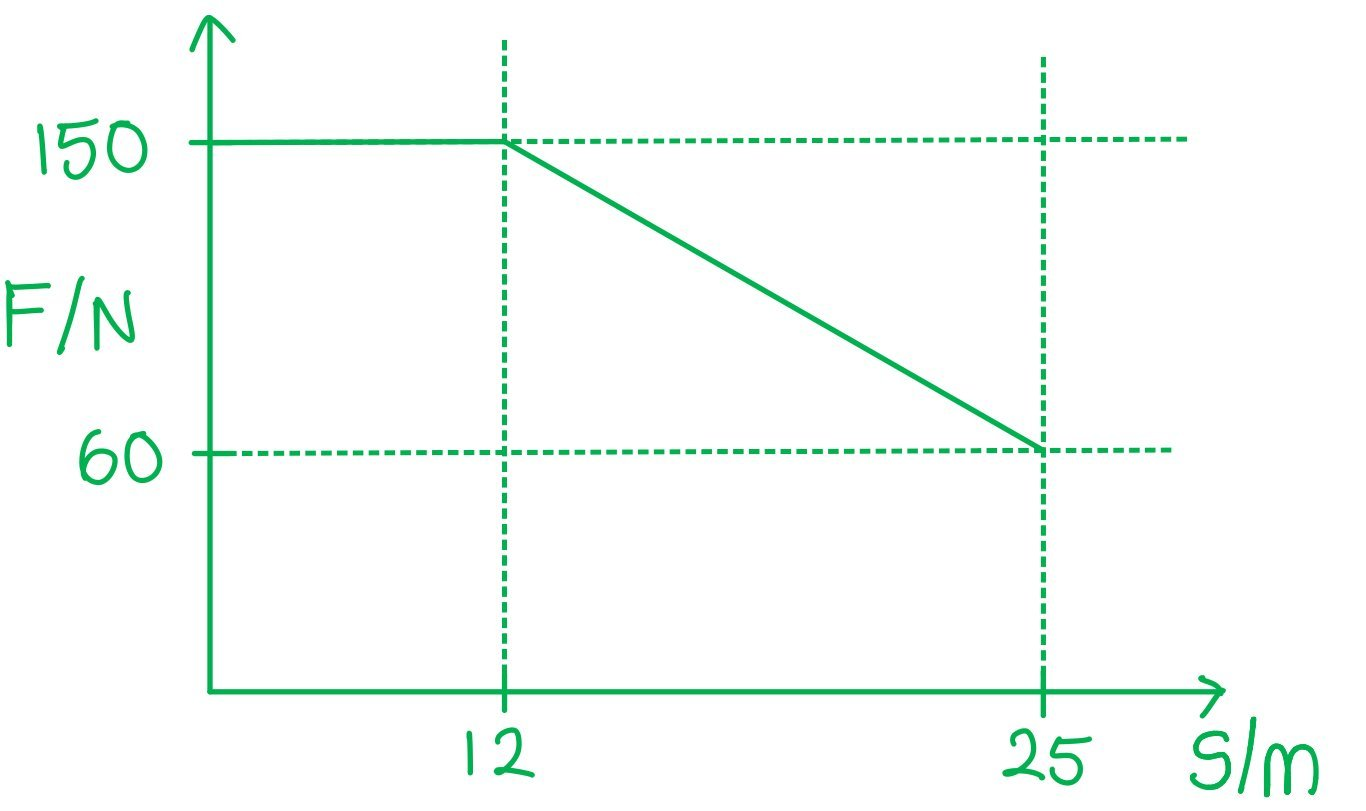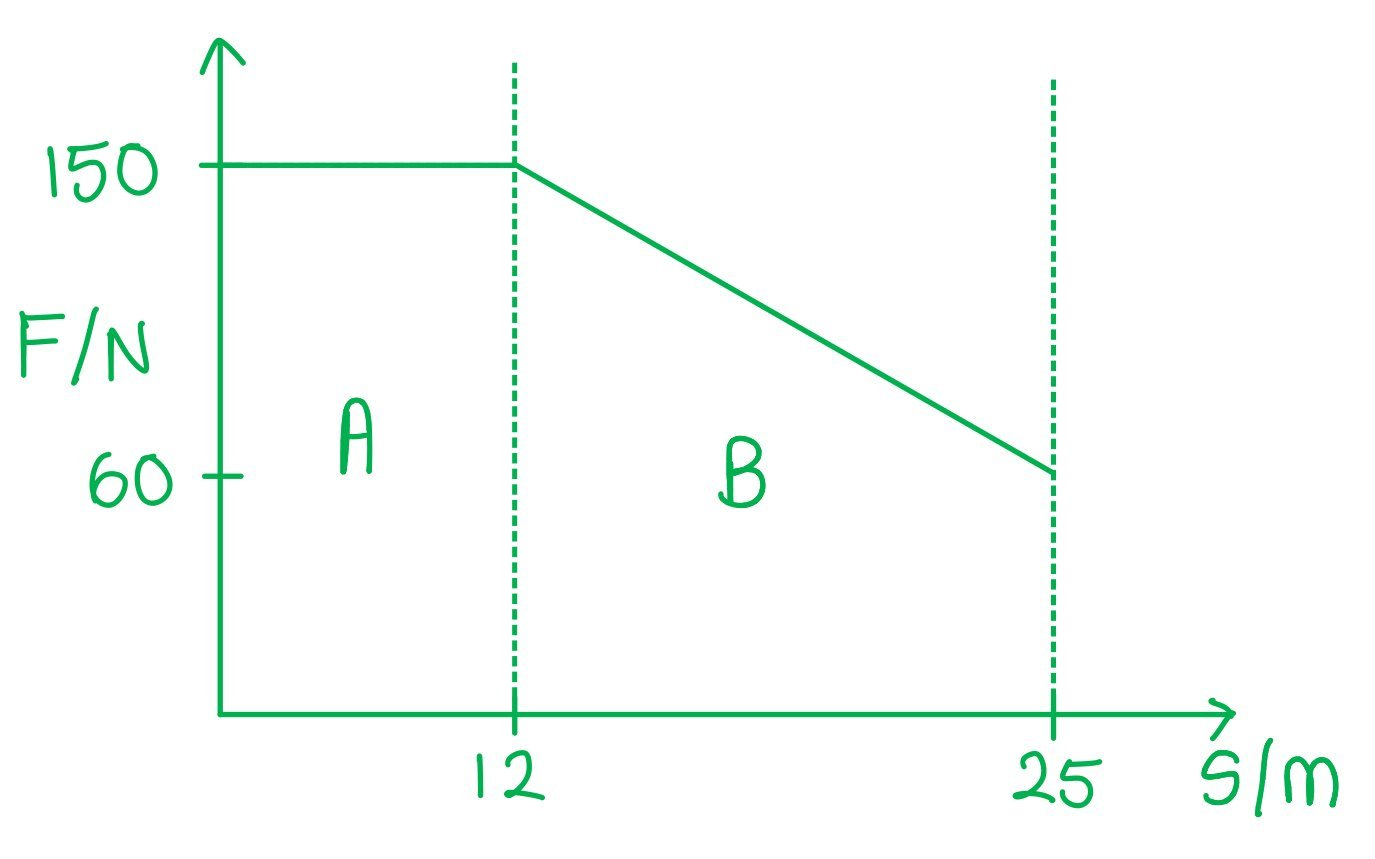# AQA A Level Physics复习笔记4.6.2 Area Under a Force-Displacement Graph

### Area Under a Force-Displacement Graph

• The work done can also be found from a force-displacement graph
• If the force is not constant and is plotted against the displacement of the object:
• The work done is equal to the area under the force-displacement graph

• This is because:

Work done = Force × Displacement

• The work done is therefore equivalent whether there is:
• A small force over a long displacement
• A large force over a small displacement

• The graph may need to be split up into sections. The total area is the sum of the areas of each sectionThe area underneath the force-displacement graph is the work done

#### Worked Example

The graph shows how a force varies over a displacement of 80 m.Calculate the work done.

Step 1: Split the graph into sections

The work done is the area under the graph

The total area can be found by splitting the graph into sections A and BStep 2: Calculate the area of section A

Section A is a right-angled triangle where the area is 0.5 × base × height

0.5 × 80 × (250 – 100) = 6000 J

Step 3: Calculate the area of section B

Section B is a rectangle where the area is base × height

80 × 100 = 8000 J

Step 4: Calculate the total work done

The total work done is the sum of both areas

Work done = 6000 + 8000 = 14 000 J

#### Exam Tip

Always check the units on the axes when calculating values from a graph. Sometimes the force will be given in kN or the displacement in km. These must be converted into SI units to calculate the work done in J.

### Variable Forces

• The force on an object may not always be constant, this is known as a variable force
• This is more representative of a force in real life
• If a force is constant, then the following equations can be used:

#### P = Fv

• If a force is varying, the above equations cannot be used, instead, work done must be found from the area under the force-displacement graph
• If a varying force increases, then an object’s acceleration increases and vice versa

#### Worked Example

A person is pulling a suitcase through an airport with a rough surface.They apply a force of 150 N over a distance of 12 m. Afterwards, the person gets progressively tired and the applied force is linearly reduced to 60 N. The total distance through which the suitcase has moved is 25 m.Calculate the work done by the force applied by the person over 25 m.

Step 1: Sketch a force-displacement graph and split it into sectionsStep 2: Split the graph into sections

• The work done is the area under the graph
• The total area can be found by splitting the graph into sections A and BStep 3: Calculate the area of section A

• Section A is a rectangle where the area is base × height

12 × 150 = 1800 J

Step 4: Calculate the area of section B

• Section B is a trapezium where the area isStep 5: Calculate the total work done

• The total work done is the sum of both areas

Work done = 1800 + 1365 = 3165 J

#### Exam Tip

When sketching graphs, they don’t have to be to scale. However, it is important to label the key points on the x and y-axis from the question to calculate the areas underneath the graph.